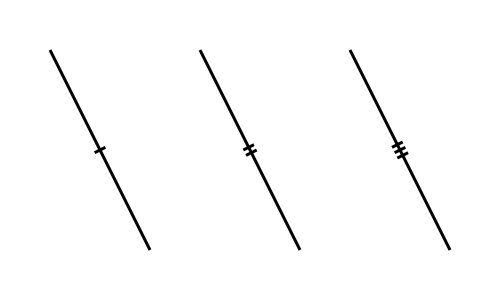# Tick

Martin McBride, 2020-08-23
Tags geometry tick
Categories generativepy generative art

The tick function adds a small cross line marker to a line, of the type often used in geometric diagrams to show that two lines are the same length. They look like this:You can add 1, 2 or 3 ticks.

## tick function

tick(ctx, a, b, count=1, length=4, gap=1)

Parameter Type Description
ctx Context The Pycairo Context to draw to
a (number, number) Tuple (x, y) for point a
b (number, number) Tuple (x, y) for point b
count int Number of ticks on the line, 1, 2 or 3.
length number length of the tick in user units.
gap number Gap between ticks in user units.

Draws an tick across the line ab. The tick is half way between points a and b.

## Example

See the example in the angle_marker article.